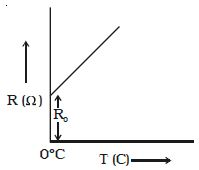Physics

# How Temperature Dependence of Resistance?

The resistivity of substances varies with temperature. For conductors the resistance increases with increase in temperature. If Ro is the resistance of a conductor at OO C and Rt is the resistance of same conductor at to C, then

Rt = Ro (1 + αt)

where α is called the temperature coefficient of resistance.

α = (Rt – Ro) / Rot

The temperature coefficient of resistance is defined as the ratio of increase in resistance per degree rise in temperature to its resistance at O0 C. Its unit is per 0C.

The variation of resistance with temperature is shown in Figure.Fig: Variation of resistance with temperature

Metals have positive temperature coefficient of resistance, i.e., their resistance increases with increase in temperature. Insulators and semiconductors have negative temperature coefficient of resistance, i.e., their resistance decreases with increase in temperature. A material with a negative temperature coefficient is called a thermistor. The temperature coefficient is low for alloys.